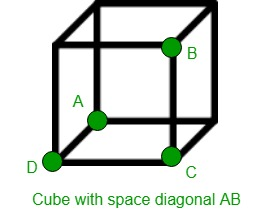GeeksforGeeks App
Open AppBrowser
Continue

# Volume of cube using its space diagonal

Given the length of space diagonal of a cube as d. The task is to calculate the volume occupied by the cube with the given length of space diagonal. Space diagonal is a line connecting two vertices that are not on the same face.Examples:

Input: d = 5
Output: Volume of Cube: 24.0563

Input: d = 10
Output: Volume of Cube: 192.45

Volume of cube whose space diagonal is given:Proof:

Let d = the length of diagonal |AB| and
let a = the length of each side of the cube.
Pythagoras #1 in triangle ACD:Pythagoras #2 in triangle ABC:Now we can solve for a in terms of d:This means that the volume V is:Below is the required implementation:

## C++

 // C++ program to find the volume occupied// by Cube with given space diagonal#include using namespace std; // Function to calculate Volumefloat CubeVolume(float d){    float Volume;     // Formula to find Volume    Volume = (sqrt(3) * pow(d, 3)) / 9;     return Volume;} // Drivers codeint main(){     // space diagonal of Cube    float d = 5;     cout << "Volume of Cube: "         << CubeVolume(d);     return 0;}

## Java

 // Java program to find the volume occupied// by Cube with given space diagonal public class GFG{         // Function to calculate Volume    static float CubeVolume(float d)    {        float Volume;             // Formula to find Volume        Volume = (float) (Math.sqrt(3) * Math.pow(d, 3)) / 9;             return Volume;    }         // Drivers code    public static void main(String []args)    {             // space diagonal of Cube        float d = 5;             System.out.println("Volume of Cube: " + CubeVolume(d));         }     // This code is contributed by Ryuga    }

## Python3

 # Python 3 program to find the volume occupied# by Cube with given space diagonalfrom math import sqrt, pow # Function to calculate Volumedef CubeVolume(d):     # Formula to find Volume    Volume = (sqrt(3) * pow(d, 3)) / 9     return Volume # Drivers codeif __name__ == '__main__':     # space diagonal of Cube    d = 5     print("Volume of Cube:",'{0:.6}' .                format(CubeVolume(d))) # This code is contributed# by SURENDRA_GANGWAR

## C#

 // C# program to find the volume occupied// by Cube with given space diagonalusing System; public class GFG{         // Function to calculate Volume    static float CubeVolume(float d)    {        float Volume;             // Formula to find Volume        Volume = (float) (Math.Sqrt(3) * Math.Pow(d, 3)) / 9;             return Volume;    }         // Drivers code    public static void Main()    {             // space diagonal of Cube        float d = 5;             Console.WriteLine("Volume of Cube: {0:F4}" , CubeVolume(d));         }     // This code is contributed by mits    }

## PHP

 

## Javascript

 

Output:

Volume of Cube: 24.0563

Time Complexity: O(1)

Auxiliary Space: O(1)

My Personal Notes arrow_drop_up